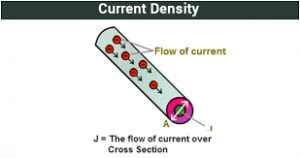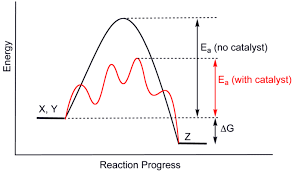## How to Calculate and Solve for Current Density | CorrosionThe image above represents current density.

To compute for current density, three essential parameters are needed and these parameters are Number of Electron Charge Present (z), Force (F) and Rate of Heterogeneous Reaction (V).

The formula for calculating current density:

j = zFV

Where:

j = Current Density
z = Number of Electron Charge Present
F = Force
V = Rate of Heterogeneous Reaction

Let’s solve an example;
Find the current density when the number of electron charge present is 6, the force is 4 and the rate of heterogeneous reaction is 8.

This implies that;

z = Number of Electron Charge Present = 6
F = Force = 4
V = Rate of Heterogeneous Reaction = 8

j = zFV
j = (6) (4) (8)
j = 192

Therefore, the current density is 192 A/m².

Calculating the Number of Electron Charge Present when the Current Density, the Force and the Rate of Heterogeneous Reaction is Given.

z = j / FV

Where;

z = Number of Electron Charge Present
j = Current Density
F = Force
V = Rate of Heterogeneous Reaction

Let’s solve an example;
Find the number of electron charge present when the current density is 40, the force is 2 and the rate of heterogeneous reaction is 4.

This implies that;

j = Current Density = 40
F = Force = 2
V = Rate of Heterogeneous Reaction = 4

z = j / FV
z = 40 / 2 x 4
z = 40 / 8
z = 5

Therefore, the number of electron charge present is 5.

## How to Calculate and Solve for Rate of Heterogeneous Reaction | CorrosionThe image above represents rate of heterogeneous reaction.

To compute for rate of heterogeneous reaction, two essential parameters are needed and these parameters are dn/dt and Amount of Material (n).

The formula for calculating rate of heterogeneous reaction:

V = dn/dt / n

Where:

V = Rate of Heterogeneous Reaction
dn/dt = dn/dt
n = Amount of Material

Let’s solve an example;
Find the rate of heterogeneous reaction when the dn/dt is 36 and the amount of material is 12.

This implies that;

dn/dt = dn/dt = 36
n = Amount of Material = 12

V = dn/dt / n
V = 24 / 12
V = 2

Therefore, the rate of heterogeneous reaction is 2.

Calculating the dn/dt when the Rate of Heterogeneous Reaction and the Amount of Material is Given.

dn/dt = V x n

Where;

dn/dt = dn/dt
V = Rate of Heterogeneous Reaction
n = Amount of Material

Let’s solve an example;
Find the dn/dt when the rate of heterogeneous reaction is 28 and the amount of material is 2.

This implies that;

V = Rate of Heterogeneous Reaction = 28
n = Amount of Material = 2

dn/dt = V x n
dn/dt = 28 x 2
dn/dt = 56

Therefore, the dn/dt is 56.